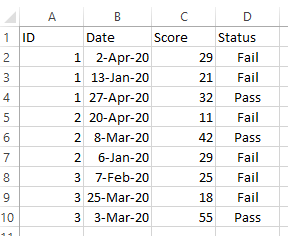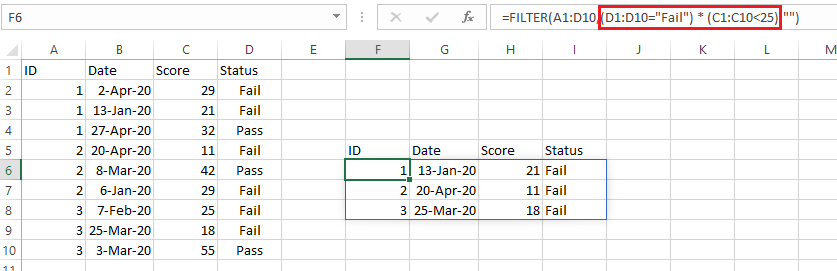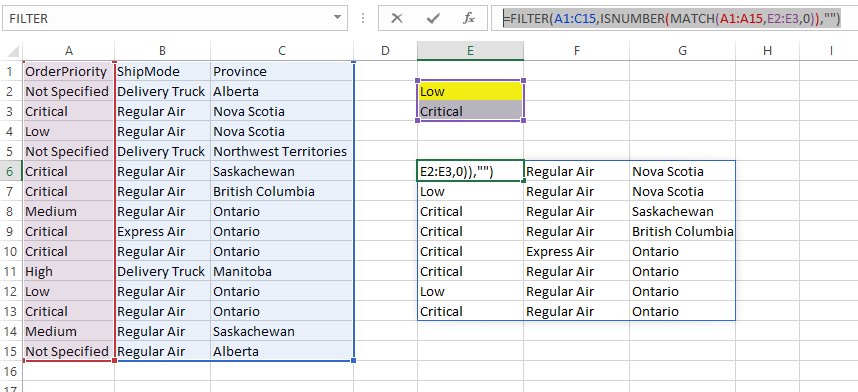Search

# How to FILTER in Excel formulas?In my early days of MS Excel, VLOOKUP was quite popular. It used to be a proud arsenal for one who is used Excel in their day-to-day work. But at times, that wasn’t enough. We wished if we could add additional filters to the VLOOKUP function. The technology was not ready then.

The past few years have been quite exciting. Microsoft has made Excel a versatile package: storing, processing & modeling data has become a more straightforward task. Inclusion of new functions, Power Query, Power Pivot, and new graphs like Box Plots, Histograms, Tree Maps, and more have made Excel quite formidable application. And finally, we have a function that allows us to include additional filters.

## Introducing FILTER

Quite a simple function, FILTER function has the following syntax:

FILTER (array, include,[if empty])

· Array: The range which we need in return

· Include: The column which we would like to filter

· [if empty] (Optional): Values to return if no matches found

The output of the FILTER function is an array that meets the criteria defined under the “Include” argument.

Let us consider the example below:We can use the following formula in cell F5

`=FILTER(A1:D10,D1:D10=F3,"")`And it returns a table with the matching Status (taking reference from cell F3)

Gif image

FILTER function can also return a single column:

`=FILTER(C1:C10,D1:D10=F3,"")`And it returns a table with the matching Status (taking reference from cell F3)FILTER function can also return a single column:

`=FILTER(C1:C10,D1:D10=F3,"")`## FILTER with AND criteria

We can combine multiple conditions using the special character “* “ in the Include argument:

`=FILTER(A1:D10,(D1:D10="Fail") * (C1:C10<25),"")`

It returns an array where the Status = Fail AND Score < 25## FILTER with OR criteria

Let us consider the example below:The aim is to return an array where the Order Priority is either Critical OR Low

To extract the required result, we can use MATCH function with FILTER:

`=FILTER(A1:C15,ISNUMBER(MATCH(A1:A15,E2:E3,0)),"")`Alternatively, we could write the same formula as:

`=FILTER(A1:C15,ISNUMBER(MATCH(A1:A15,{"Critical","Low"},0)),"")`

Using * operator, we can combine multiple OR criteria:

`=FILTER(A1:C15,ISNUMBER(MATCH(A1:A15,E2:E3,0)) * ISNUMBER(MATCH(C1:C15,G2:G3,0)),"")`## Using FILTER with VLOOKUP & XLOOKUP

FILTER function is quite useful when used with VLOOKUP or XLOOKUP. We can return the search result by meeting multiple criteria.

`=VLOOKUP(F4,FILTER(A1:D10,D1:D10 = "Pass",""),3,0)`

` =XLOOKUP(F4,FILTER(A1:A10,D1:D10="Pass",""),FILTER(C1:C10,D1:D10="Pass",""))`This formula returns the result based on ID and Status = "Pass"

@imVivRan